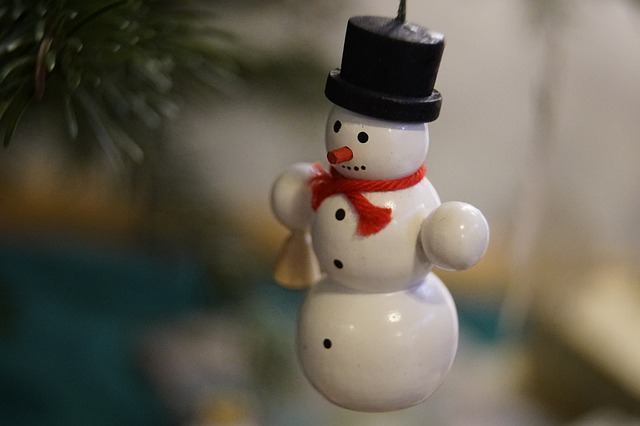#### You may also like### Overturning Fracsum

Can you solve the system of equations to find the values of x, y and z?### Network Trees

Explore some of the different types of network, and prove a result about network trees.### Always Two

Find all the triples of numbers a, b, c such that each one of them plus the product of the other two is always 2.

# A Frosty Puddle

##### Age 16 to 18Challenge LevelIf you are getting stuck on this question, start by reading A Guide to Problem Solving.  You can also use the Getting Started tab, where you will find some hints and tips to guide you through the question.

This problem follows on from Frosty the Snowman.

Frosty the snowman is made from two uniform spherical snowballs, of initial radii $2R$ and $3R.$ The smaller (which is his head) stands on top of the larger.

As each snowball melts, its volume decreases at a rate which is directly proportional to its surface area, the constant of proportionality being the same for both snowballs. During melting each snowball remains spherical and uniform.

Let $V$ and $h$ denote Frosty's total volume and height at time $t$.

• Show that, for $2R <h \le 10R, \qquad$ $\dfrac{\mathrm{d} V}{\mathrm{d} h}=\frac{\pi} 8 (h^2 + 4R^2)$

• Derive the corresponding expression for $0 \le h < 2R$

• Sketch $\dfrac{\mathrm{d} V}{\mathrm{d} h}$ as a function of $h$ for $4R \ge h \ge 0$.  Hence give a rough sketch of $V$ as a function of $h$.

This question has been adapted and changed several times since it was first published.  You can see some of the various versions via the Teachers Resources tab.

Adapted from STEP Mathematics I, 1991, Q2. Question reproduced by kind permission of Cambridge Assessment Group Archives. The question remains Copyright University of Cambridge Local Examinations Syndicate ("UCLES"), All rights reserved.